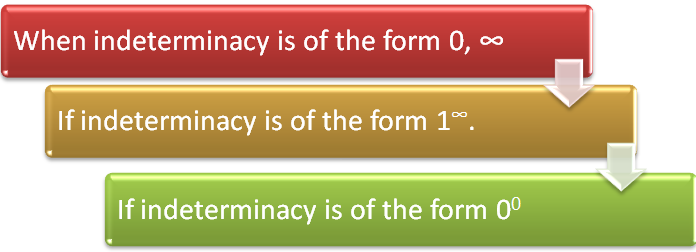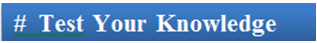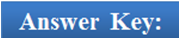×#### Thank you for registering.

One of our academic counsellors will contact you within 1 working day.

Click to Chat

1800-1023-196

+91-120-4616500

CART 0

• 0

MY CART (5)

Use Coupon: CART20 and get 20% off on all online Study Material

ITEM
DETAILS
MRP
DISCOUNT
FINAL PRICE
Total Price: Rs.

There are no items in this cart.
Continue Shopping```Limits using DifferentiationL'Hospital's RuleWhile computing limits, we often encounter situations, where the limits take one of the following forms  0/0,∞/∞,∞ - ∞,0 × ∞ and 1-, 0-, ∞o. is it possible to compute the limit of functions in such cases?

These values are termed as indeterminate values in the language of mathematics. In fact, with just the knowledge of limits one cannot expect to solve such limits. The rule used for computing the limits of indeterminate forms is termed as L’Hospital’s Rule.

If f(x) and g(x) are differential functions of x and

1. If f(a) = 0 and g(a) = 0 or f(a) = ∞ and g(a) = ∞ then

lim (x→a) (f(x))/(g(x)) = lim(x→a) (f' (x))/(g' (x))

In general if f(a) = f'(a) – f"(a) = .............. fn-1(a) = 0 (or ∞)

And g'(a) = g"(a) = ........................ = gn-1(a) = 0 (or ∞)

Where fn(a) ≠ 0 (or ∞) and gn(a) ≠ 0, then

lim (x→a) (f(x))/(g(x)) = lim (x→a) (fn (x))/(gn (x)).Note: L 'Hospital's rule is applicable only when the given expression is of the form 0/0, ∞/∞.

Let us have a look at some of the illustrations involving limits of the form 0/0 or ∞/∞:

Illustration 1:

Evaluate lim (x→0) (ex sin x – x – x2)/(x2 + x – ln (1-x))

Solution:

S = lim (x→0) (ex sin x – x – x2)/(x2 + x – ln (1 – x)) (0/0 form)

= lim (x→0) [ex (cos x + sin x) – 1 – 2x] / [ 2x + 1 + 1/(1 – x)] (0/0 form)

= (1 – 1 – 0)/(0 + 1 + 1)

= 0.

_________________________________________________________________

Illustration 2:

Evaluate lim (x→∞) (2x2 + 3x – 1)/(3x2 - 5x + 2).

Solution:

The given expression is lim(x→0) (2x2 + 3x – 1)/(3x2 - 5x + 2)

= lim (x→∞) (2x2 + 3x – 1)/(3x2 - 5x + 2) (∞/∞ form)

= lim (x→∞) (4x + 3)/(6x – 5) (differentiating numerator and denominator)

= lim (x→∞) 4/6

= 2/3.

____________________________________________________________________

Illustration 3:

lim(x→1) (x7 - 2x5 + 1)/(x3 - 3x2 + 2)

Solution:

The question can be solved by two methods:

Method 1: lim(x→1) (x7 - 2x5 + 1)/(x3 - 3x2 + 2) [0/0 form]

Using L'Hospital’s rule we can get the value of limit by differentiating the denominator and numerator separately.

lim (x→1) (7x6 - 10x4)/(3x2 - 6x)

= (7 – 10)/(3 – 6) = 1

Method 2: Alternatively, because (x – 1) factor is common to both Numerator and Denominator, hence we can write,

lim (x→1) (x7 - 2x5 + 1)/(x3 - 3x2 + 2) = lim (x→1) [x6(x-1) + x5(x-1) -  (x5-1)]/[(x2(x-1) - 2(x2 -1)]

= lim (x→1) [(x-1) (x6 + x5 – (x4 + x3 + x2 + 1)]/[(x-1) (x2 - 2x - 2)]

=  lim (x→1) [x6 + x5 - x4 - x3 - x2 - 1)]/[(x2 - 2x - 2)

= (1 + 1 – 1 – 1 – 1 – 1 – 1)/(1 – 2.1 – 2)

= 1.

Remark:

If the problem is not in the form of 0/0 or ∞/∞, we can simplify the expression till it becomes of the form 0/0 or ∞/∞. Hence, any form of indeterminacy can always be expressed in the form 0/0 or ∞/∞ which can then be easily solved by applying the L Hospital’s Rule. The chart given below illustrates the various possible forms of indeterminacy and how they can be modified so as to apply the Hospital’s Rule:Any form of indeterminacy can always be put in the form 0/0 and ∞/∞ so that we can then solve it by applying the L' Hospital’s Rule. Let us see how L' Hospital’s Rule can be applied in the following cases:

Case I: When indeterminacy is of the form 0, ∞

Let lim (x→a) f(x) g(x) = 0, ∞ (f(a) = 0, g(a) = ∞)

The expression can be modified as

lim (x→a) f(x)/(1 ⁄ (g(x))) = 0/0 form and L' Hospital Rule can be applied.

Illustration:

Find lim (x→π/2) (π/2 – x) tan x

lim (x→π/2) (π/2 – x) tan x              [0.∞ form]

= lim (x→π/2) (π/2 – x)/cot x           [0/0 form]

= lim (x→π/2) – (0 – 1)/cosec2 x = lim (x→π/2) sin2 x

= 12

= 1.

Refer the following video for more on L Hospital’s Rule

Case II: If indeterminacy is of the form 1∞.

Let y = lim (x→a) [f(x)]g(x) is of the form 1∞

Then we can write

loge y = lim (x→a) g(x) loge f(x) (now form ∞ × 0 since loge 1 = 0)

= lim (x→a) log f(x)/(1 ⁄ (g(x))) (now 0/0 form)

By applying the L' Hospital Rule one gets a value for the limit 'a' (say)

Then we have log y = α

or y = eα.

Case III: If indeterminacy is of the form 00

y = lim (x→a) [f(x)](g(x)) is of the form 00.

then expression can be written as

log y = lim (x→a) g(x) log f(x) (Now form 0 × - ∞ since loge0 = -∞)

and expression takes the form

log y = lim (x→a) g(x)/(1 ⁄ log f(x) ) (form 0/0)

By using L' Hospital Rule one can evaluate this limit.Q1. L Hospital’s Rule is used for finding the

(a) determinate limits

(b) Indeterminate limits

(c) differential coefficient

(d) none of these

Q2. If indeterminacy is of the form 1∞

(a) we can determine the limit using L Hospital’s rule after taking logarithm of the function.

(b) the limit can’t be determined.

(c) the limit can be determined without using the L Hospital’s Rule.

(d) none of these.

Q3. If f(a) = 0 and g(a) = 0 or f(a) = ∞ and g(a) = ∞ then

(a) lim (x→a) (f(x))/(g(x)) = lim (x→a) (f' (x))/(g(x))

(b) lim (x→a) (f(x))/(g(x)) = lim (x→a) (f(x))/(g' (x))

(c) lim (x→a) (f(x))/(g(x)) = lim (x→a) (f' (x))/(g' (x))

(d) none of these

Q4. L 'Hospital's rule is applicable only when the given expression is

(a) of the form 1/1.

(b) can be of any form.

(c) of the form 0/0, ∞/∞.

(d) of the form 0/0, ∞/∞ or is reducible to one of these forms.Q1.

Q2.

Q3.

Q4.

(b)

(a)

(c)

(d)

Related Resources

For getting an idea of the type of questions asked, refer the previous year papers.

Fast Track your revision with the ultimate Revision Notes on Trigonometry.

To read more, Buy study materials of Methods of Differentiation comprising study notes, revision notes, video lectures, previous year solved questions etc. Also browse for more study materials on Mathematics here.
```### Course Features

• 731 Video Lectures
• Revision Notes
• Previous Year Papers
• Mind Map
• Study Planner
• NCERT Solutions
• Discussion Forum
• Test paper with Video Solution